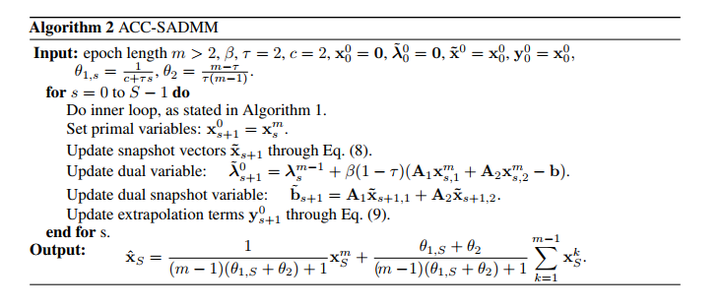# Faster and Non-ergodic O(1/K) Stochastic Alternating Direction Method of Multipliers### Abstract

We study stochastic convex optimization subjected to linear equality constraints. Traditional Stochastic Alternating Direction,Method of Multipliers and its Nesterov’s acceleration scheme can only achieve ergodic O(1/√K) convergence rates, where K is the number of iteration. By introducing Variance Reduction (VR) techniques, the convergence rates improve to ergodic O(1/K). In this paper, we propose a new stochastic ADMM which elaborately integrates Nesterov’s extrapolation and VR techniques. With Nesterov’s extrapolation, our algorithm can achieve a non-ergodic O(1/K) convergence rate which is optimal for separable linearly constrained non-smooth convex problems, while the convergence rates of VR based ADMM methods are actually tight O(1/√K) in non-ergodic sense. To the best of our knowledge, this is the first work that achieves a truly accelerated, stochastic convergence rate for constrained convex problems. The experimental results demonstrate that our algorithm is faster than the existing state-of-the-art stochastic ADMM methods.

Type
Publication
Neural Information Processing Systems